# Fundamental group of wedge sum relative to basepoints with neighborhoods that deformation retract to them is free product of fundamental groups

Jump to: navigation, search

## Statement

Suppose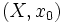$(X,x_0)$ and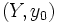$(Y,y_0)$ are based topological spaces. Suppose, further, that$X$ and$Y$ are both path-connected spaces (otherwise, we basically care only about the path components of$X$ and$Y$). Consider the Wedge sum (?):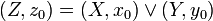$\! (Z,z_0) = (X,x_0) \vee (Y,y_0)$

Here,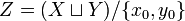$Z = (X \sqcup Y)/\{ x_0, y_0 \}$ and the identified point$\! x_0 \sim y_0$ is labeled$z_0$.

Suppose, further that:

• There exists an open subset$A$ of$X$ containing$x_0$ such that$A$ has a strong deformation retraction to$x_0$.
• There exists an open subset$B$ of$Y$ containing$y_0$ such that$B$ has a strong deformation retraction to$y_0$.

Then, we have the following relationship between the fundamental groups of$(X,x_0)$,$(Y,y_0)$, and$(Z,z_0)$: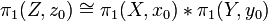$\! \pi_1(Z,z_0) \cong \pi_1(X,x_0) * \pi_1(Y,y_0)$

where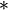$\! *$ denotes the free product of groups.

## Facts used

1. Seifert-van Kampen theorem